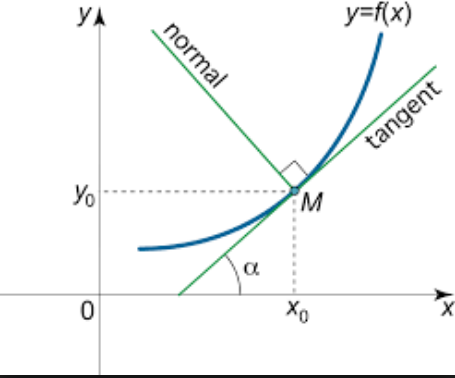Filters

Q&A - Ask Doubts and Get Answers
Q

Give answer! Equation of curves such that tangent and normal drawn at any point of which are of equal length, is

Equation of curves such that tangent and normal drawn at any point of which are of equal length, is

• Option 1)

• Option 2)

• Option 3)

• Option 4)

82 Views

As we have learnt,

Length of Normal -

- whereinSquaring both sides we have

Curves are either

Option 1)

Option 2)

Option 3)

Option 4)

Exams
Articles
Questions